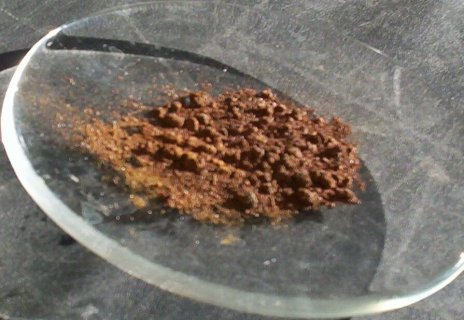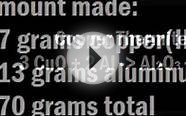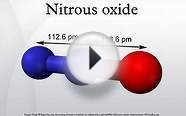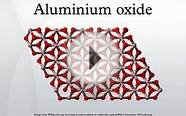# Copper I oxide chemical formula

The procedure that can be followed when confronted with the name of a compound and you wish to write its formula is as follows:

3. Balance the total positive and negative charge on the cation and anion. You ask yourself do the total positive charge and total negative charge add up to zero. If the answer is no then we ask how many of each ion must we have in order to balance charge. We must have the same number of positive charges as we do of negative charges. Another way of saying that is that they must add up to zero.

4. Once you have determined the number of units of the cation and anion those become the subscripts which are placed right after the respective symbol.

Identify the symbols of the cation and anion

Copper is Cu and Oxide is O

Identify the charge for each and place above the symbol in parenthesis

For Copper I that would be 1+ and for Oxide that would be 2-

Balance the positive and negative charges

Since each Copper is 1+ and each Oxide is 2- then it will take two Cu+ to balance one oxide with a 2- so that

2(1+) + 1(2-) = 0. The numbers outside the parenthesis become the subscripts in the formula

Write the formula placing the subscripts right after the symbol they go with.

Cu2O

Identify the symbols of each part of the name

Calcium symbol is Ca and Nitride symbol is N

Identify the charge for each

Calcium belongs from Group 2 which always has a +2 and Nitride will be a single Nitrogen with a -3 charge

Balance charge

Since Calcium is +2 and Nitride is -3 the only way to balance them is to have three Calcium's and two nitrides

Write the symbol beginning with the symbol that is first in the name and include the subscript after each symbol

Ca3N2

Formula writing with Polyatomic Ions

1. Identify the symbol of the cation (first part of the name) and the anion

The symbol for Iron is Fe and the symbol for Carbonate which is a polyatomic ion is CO3

2. Identify the valence or charge of each symbol and place it in parenthesis just above the symbol

The valence for Iron (III) is 3+ and the valence for Carbonate is 2-

3. Balance the total positive and negative charge on the cation and anion. You ask yourself do the total positive charge and total negative charge add up to zero. If the answer is no then we ask how many of each ion must we have in order to balance charge. We must have the same number of positive charges as we do of negative charges. Another way of saying that is that they must add up to zero. Since an Iron (III) has a +3 charge and the Carbonate ion has a 2- then it would take two Fe3+ units to balance three CO32- unitsATTITUDE Bathroom / Mold and Mildew Cleaner - 27.1 oz Home (Bio-Spectra) Certified EcoLogo, an Environment Canada program CO2 neutral: no contribution to climate change Free of petrochemical derivatives Vegan product. Vegetable-based / Natural / Biodegradable Free of chlorine, ethylene oxide and 1,4-dioxane